## Fraction of a Set

Practice Unlimited Questions

#### 1. There are 7 fowls. 4 out of the 7 fowls are chickens. What fraction of the fowls are chickens?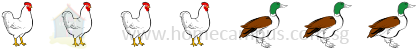4 7
of the fowls are chickens.

#### 2. There are 21 fowls. 4 7 of the fowls are chickens. How many chickens are there?

Since the denominator is 7, divide the fowls into 7 equal groups.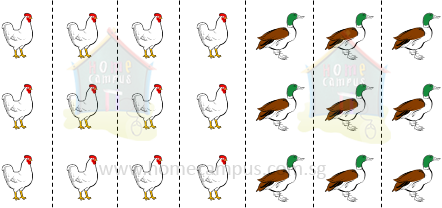4 7
of 21 = 12
There are 12 chickens.

#### 3. What fraction of the set in each of the following is shaded?

a.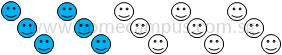The fraction of the set that is shaded  =
 2 5
b.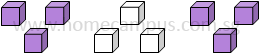The fraction of the set that is shaded  =
 2 3
c.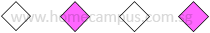The fraction of the set that is shaded  =
 2 4
=
 1 2
d.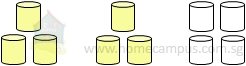The fraction of the set that is shaded  =
 6 10
=
 3 5

#### 4. Find the value of each of the following:

a.
 5 6
of 18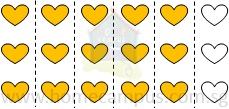Divide 18 into 6 equal groups.
Five groups are
 5 6
of 18.

 5 6
of 18
 5 6
×  18
 5 x 18 6

 90 6

=   15
b.
 1 5
of 20

 1 5
of 20
 1 5
×  20
 1 x 5 20

 5 20

=     4
c.
 1 4
of 7

 1 4
of 7
 1 4
×  7
 1 x 7 4

=
 7 4

=     7 ÷ 4
=     1.75

#### 5. There were 10 children in the playground. 4 of them were playing hopscotch. What fraction of the children were not playing hopscotch? Give your answer in its simplest form.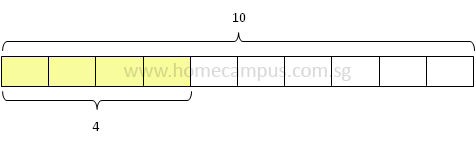6 out of 10 children were not playing hopscotch.
 6 10
=
 3 5

 3 5
of the children were not playing hopscotch.